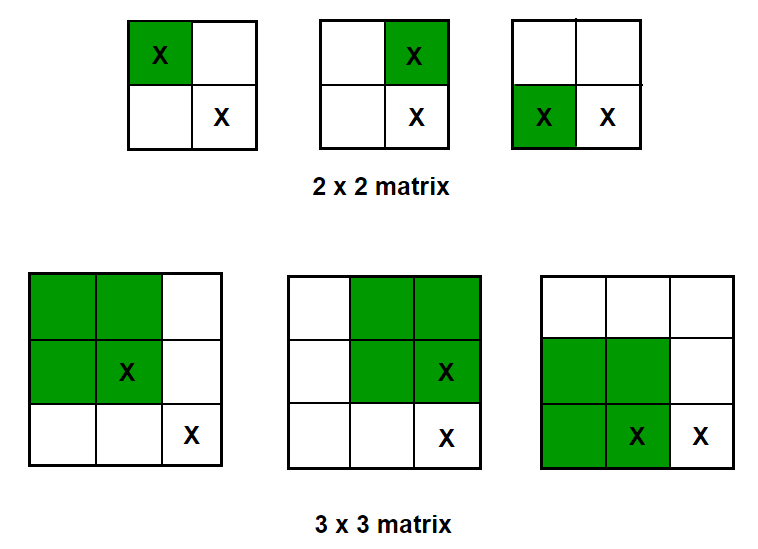# Find size of largest square sub-matrix of 1’s present in given binary matrix

Given a M x N binary matrix, find the size of largest square sub-matrix of 1’s present in it.

For example, the size of largest square sub-matrix of 1’s is 3 in below matrix:

0   0   1   0   1   1
0   1   1   1   0   0
0   0   1   1   1   1
1   1   0   1   1   1
1   1   1   1   1   1
1   1   0   1   1   1
1   0   1   1   1   1
1   1   1   0   1   1

The idea is to use Dynamic Programming to solve this problem. The problem has an optimal substructure. The size of largest square sub-matrix ending at a cell M[i][j] will be 1 plus minimum among largest square sub-matrix ending at M[i][j-1], M[i-1][j] and M[i-1][j-1]. The result will be the maximum of all square sub-matrix ending at M[i][j] for all possible values of i and j.

How does this works?

Let us consider any 2×2 matrix. In order for it to be a 2×2 matrix, each of top, left, and top-left neighbor of its bottom-right corner has to be a 1×1 square matrix.

Similarly for a 3×3 matrix, each of top, left, and top-left neighbor of its bottom-right corner has to be a 2×2 square matrix.

In general for any n x n square matrix, each of its neighbors at the top, left, and top-left corner should at-least have size of (n-1)x(n-1). The reverse of this statement is also true. If the size of the square sub-matrix ending at top, left, and top-left neighbors of any cell in the given matrix is at-least (n-1), then we can get n x n sub-matrix from that cell. That is the reason behind picking up the smallest neighboring square and adding 1 to it.

Below figure might help in visualizing things better –Below is C++ and Java implementation of the idea:

## C++

Output:

The size of largest square sub-matrix of 1’s is 3

## Java

Output:

The size of largest square sub-matrix of 1’s is 3

The time complexity of above solution is exponential and auxiliary space used by the program is O(1).

Above solution exhibits overlapping subproblems. If we draw the recursion tree of the solution, we can see that the same sub-problems are getting computed again and again. We know that problems having optimal substructure and overlapping subproblems can be solved by using dynamic programming, in which subproblem solutions are memoized rather than computed again and again. The Memoized version follows the top-down approach, since we first break the problem into subproblems and then calculate and store values. We can also solve this problem in bottom-up manner. In the bottom-up approach, we solve smaller sub-problems first, then solve larger sub-problems from them.

Below is C++ and Java implementation of the idea:

## C++

Output:

The size of largest square sub-matrix of 1’s is 3

## Java

Output:

The size of largest square sub-matrix of 1’s is 3

The time complexity of above solution is O(M x N) and auxiliary space used by the program is O(M x N).

Exercise: Find the size of largest rectangular sub-matrix of 1’s present in given binary matrix.(4 votes, average: 5.00 out of 5)Loading...

Please use our online compiler to post code in comments. To contribute, get in touch with us.
Like us? Please spread the word and help us grow. Happy coding 🙂Subscribe
Notify ofGuest

Best articleGuest

Great article. But one little issue. Your naive solution doesn’t work if the bottom right element is 0 i.e. MAT[M-1][N-1] = 0.Guest

Thx for the article ……!
But there is one bug in the recursive code
For the input :-
int[][] M =
{
{ 0, 0, 1 },
{ 0, 0, 0 },
{ 0, 0, 0 }
};
it gives answer : 0
correct ans : 1

the issue is in the base case, as we are not updating the maxSize for the base case